Get instant live expert help with Excel or Google Sheets“My Excelchat expert helped me in less than 20 minutes, saving me what would have been 5 hours of work!”

#### Post your problem and you’ll get expert help in seconds.

Your message must be at least 40 characters
Our professional experts are available now. Your privacy is guaranteed.

# How to Multiply in Excel and Google Sheets

The basic function of Excel is to manipulate the numbers to perform different calculations using different mathematical operations. We will learn how to multiply in Excel in the following examples:

• How to multiply the cells in Excel
• How to multiply the columns in Excel
• How to multiply a column by a constant in Excel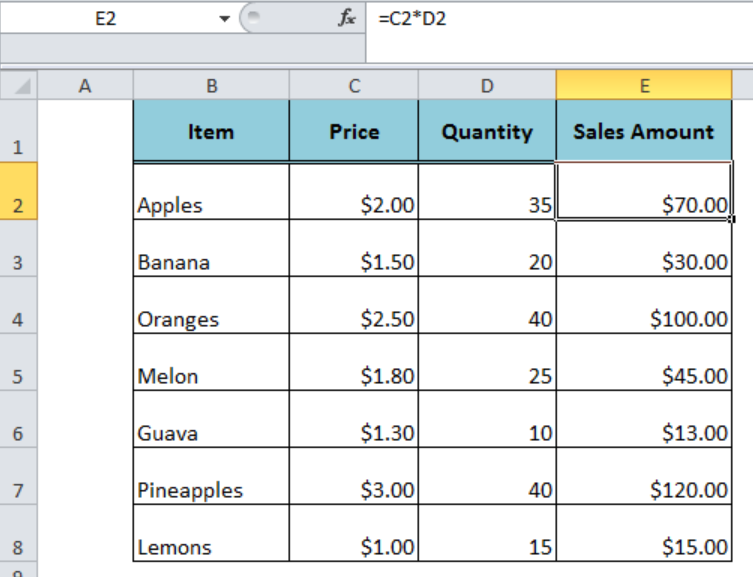Figure 1. How to Multiply in Excel and Google Sheets

## How to Multiply the Cells in Excel

We supply the cell references separated by the multiplication symbol (*) in the formula to multiply two cells in Excel, such as;

`=C2*D2`

Press Enter to get the result and copy the formula down to other cells where data exists.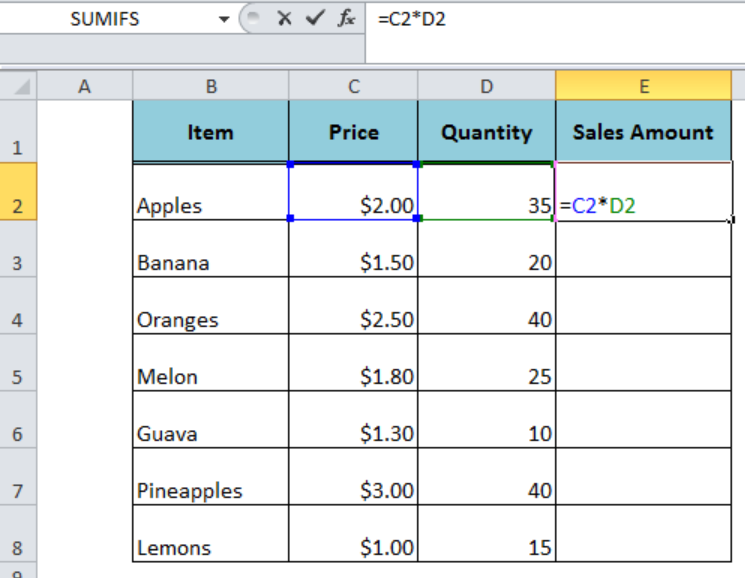Figure 2. Multiplying the Cells

## How to Multiply Columns in Excel

There are a number of ways to multiply the columns in Excel and Google Sheets.

### Multiplying One Column By Another

By using the multiplication symbol in Excel (*) we can easily multiply one column by another in Excel. Suppose we have listed the items’ prices in column C and quantities in column D and we can multiply columns C and D to get the Sales amount of each item in column E. Follow these steps;

• Insert the symbol = in column D, say row 2, and separate the column C from column D by multiplication symbol (*) to perform multiplication, such as `=C2*D2`
• Copy the formula down the column till the last cell with data.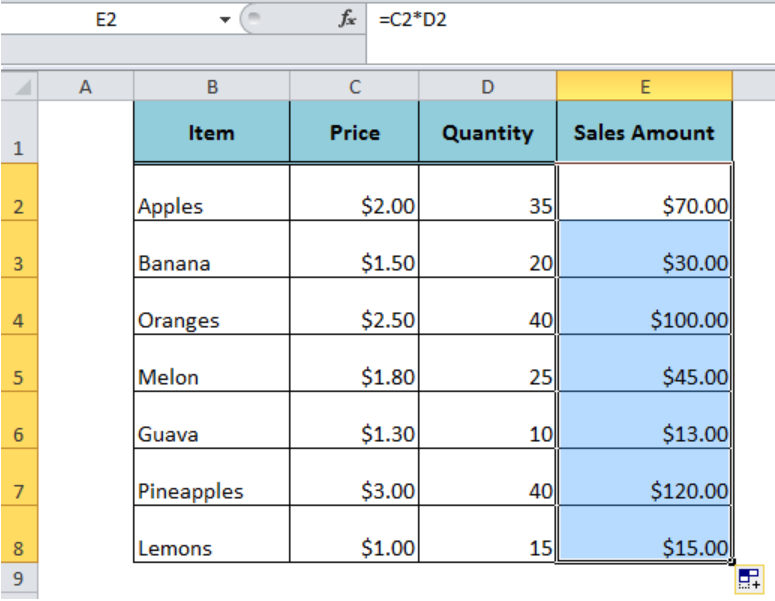Figure 3. Multiplying One Column By Another

### Multiplying Columns with PRODUCT Function

Using the PRODUCT function we can easily multiply columns in Excel and Google Sheets. In order to get the total Sales amount of price and quantity in column C and D, we use the PRODUCT function in column E, such as;

• Insert the formula `=PRODUCT(C2:D2)` in column E at row 2
• Copy this formula down to column till the last cell with data.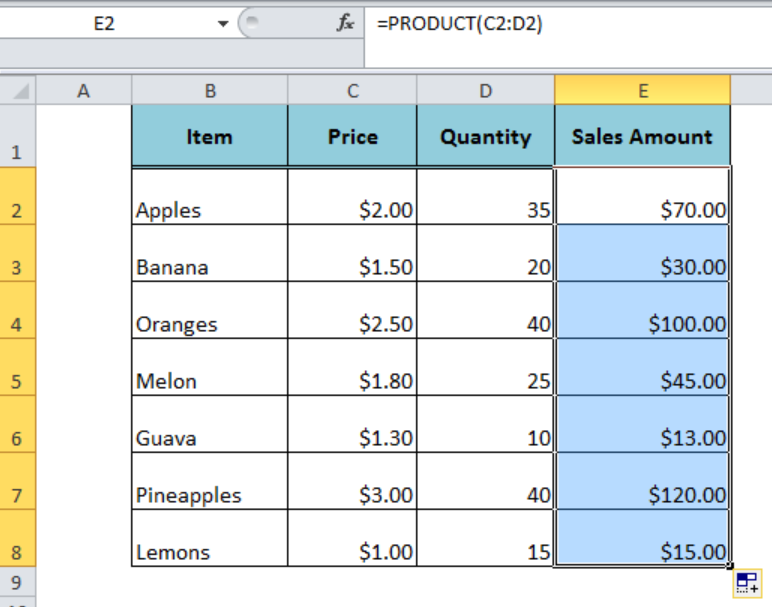Figure 4. Multiplying Columns with PRODUCT Function

### Multiplying Columns with an Array Formula

We can also multiply columns in Excel and Google Sheets with the help of an array formula. In our example, we can multiply the column C and D to get the Sales amount in column E with an array formula in the following steps;

• Select the range of cells in column E, say E2: E8, where we need to multiply columns C and D
• In the formula bar, enter the formula to multiply two columns in Excel, such as `=C2:C8*D2: D8`
• Press keys Ctrl+Shift+Enter instead of just Enter to make this formula as an array formula.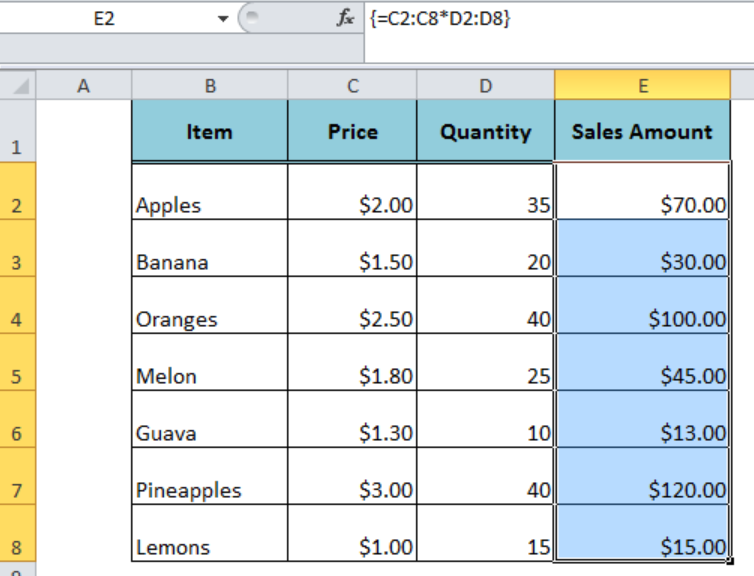Figure 5. Multiplying Columns with an Array Formula

## How to Multiply a Column By a Constant in Excel

We often need to multiply a column in Excel by a constant number or percentage value to perform calculations. We can supply the constant in the multiplication formula in Excel as a direct number or cell reference. Suppose we need to calculate VAT Amount by multiplying the Sales amount with 10% (constant) in column F.

• In column F, cell F2, enter the formula `=E2*10%`, where 10% is a constant to multiply column E.
• Copy the formula down to column E till the last cell with data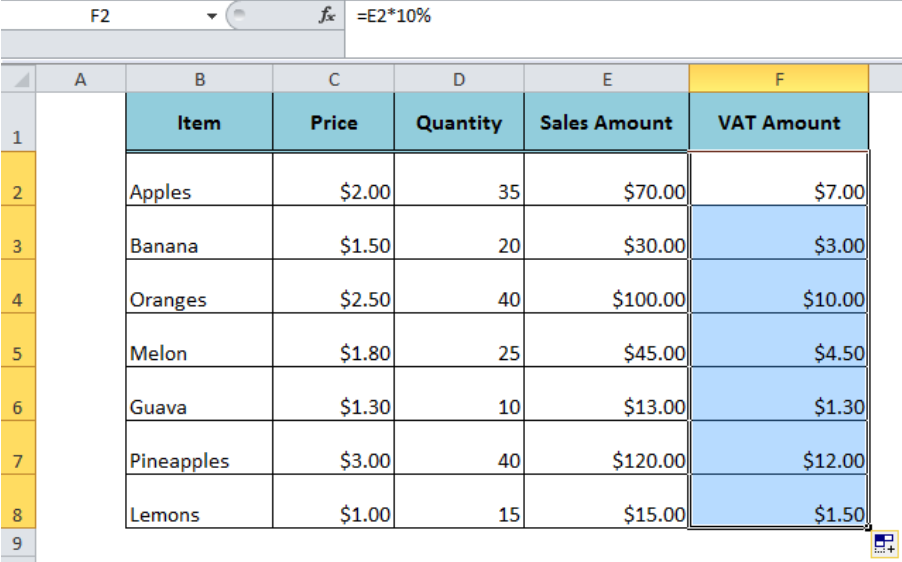Figure 6. Multiplying a Column By a Constant

Most of the time, the problem you will need to solve will be more complex than a simple application of a formula or function. If you want to save hours of research and frustration, try our live Excelchat service! Our Excel Experts are available 24/7 to answer any Excel question you may have. We guarantee a connection within 30 seconds and a customized solution within 20 minutes.

Solution examplesI want a formula which says if x =" y" or "z" or "whatever" multiple a by either "x" or "y" but get a VALUE error- any ideas? =PRODUCT(IF('Historical data'!I5="Research Associate",Costs!\$B\$18*'Historical data'!F5),PRODUCT(IF('Historical data'!I5="Senior Research Associate A",Costs!\$C\$18*'Historical data'!F5),PRODUCT(IF('Historical data'!I5="Senior Research Associate B",Costs!\$D\$18*'Historical data'!F5),PRODUCT(IF('Historical data'!I5="Freelancers",Costs!\$E\$18*'Historical data'!F5),PRODUCT(IF('Historical data'!I5="Research Director",Costs!\$F\$18*'Historical data'!F5),PRODUCT(IF('Historical data'!I5="Company Director",Costs!\$G\$18*'Historical data'!F5),PRODUCT(IF('Historical data'!I5="Managing Director",Costs!\$H\$18*'Historical data'!F5),"")))))))
Solved by V. Y. in 20 minsHi - I need a formula that can look at a Member Number (Column A) and report back if location (Column B) matches 1 of 6 locations, then looks to the date of occurance (Column N) but only adds back 1 value of repeating dates. I have this, but it is not coming back with the right answer... =SUMPRODUCT(('2017'!I\$3:I\$10000=\$A223)*ISNUMBER(SEARCH({"2","6","58","69","76","97"},'2017'!N\$3:N\$10000))) Information is corporate private, so I won't be uploading file.
Solved by S. L. in 60 minshi, i have this formula =SUMPRODUCT((B4:B464<>"")/COUNTIF(B4:B464,B4:B464&"")), i want to add a subtotal to it. so when I filter the column it also sums up the unique number if enteries i have filtered on.
Solved by F. L. in 60 minsHi, I need a formula to work out how many values are less than a certain time value, simple "countif" do not work as it is a filtered spreadsheet. Here's the formula that I have got so far: SUMPRODUCT(SUBTOTAL(3,OFFSET(Current_Month!N\$2:N\$10000,ROW(Current_Month!N\$2:N\$10000)-MIN(ROW(Current_Month!N\$2:N\$10000)),,1)),--(Current_Month!N\$2:N\$10000>"24:0:0")
Solved by K. L. in 30 mins